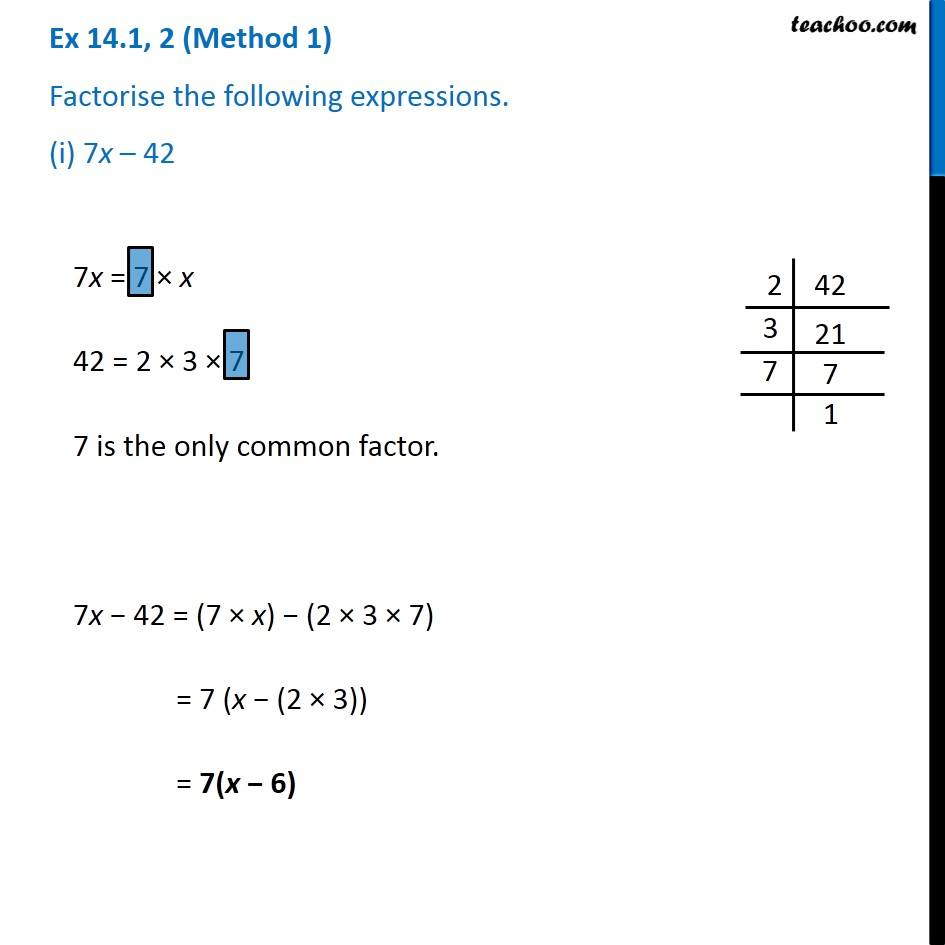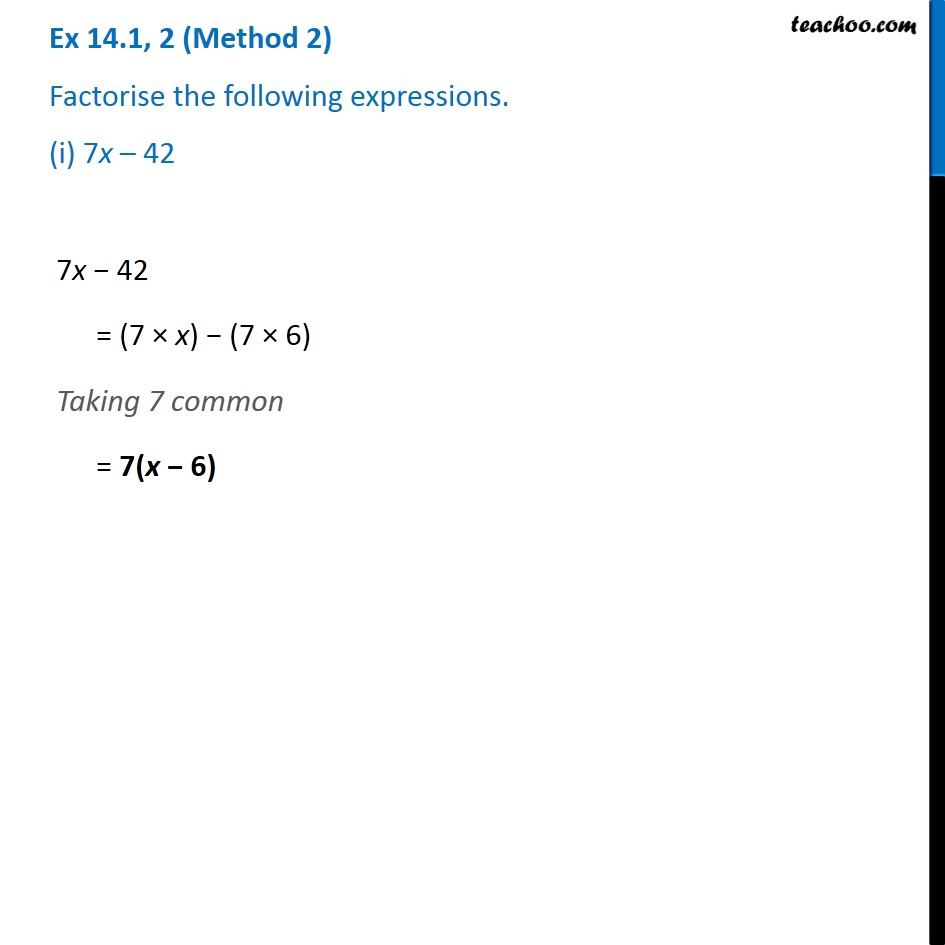Factorisation using common factors

Chapter 12 Class 8 Factorisation
Concept wiseGet live Maths 1-on-1 Classs - Class 6 to 12

### Transcript

Ex 12.1, 2 (Method 1) Factorise the following expressions. (i) 7x – 42 7x = 7 × x 42 = 2 × 3 × 7 7 is the only common factor. 7x − 42 = (7 × x) − (2 × 3 × 7) = 7 (x − (2 × 3)) = 7(x − 6) Ex 12.1, 2 (Method 2) Factorise the following expressions. (i) 7x – 42 7x − 42 = (7 × x) − (7 × 6) Taking 7 common = 7(x − 6)